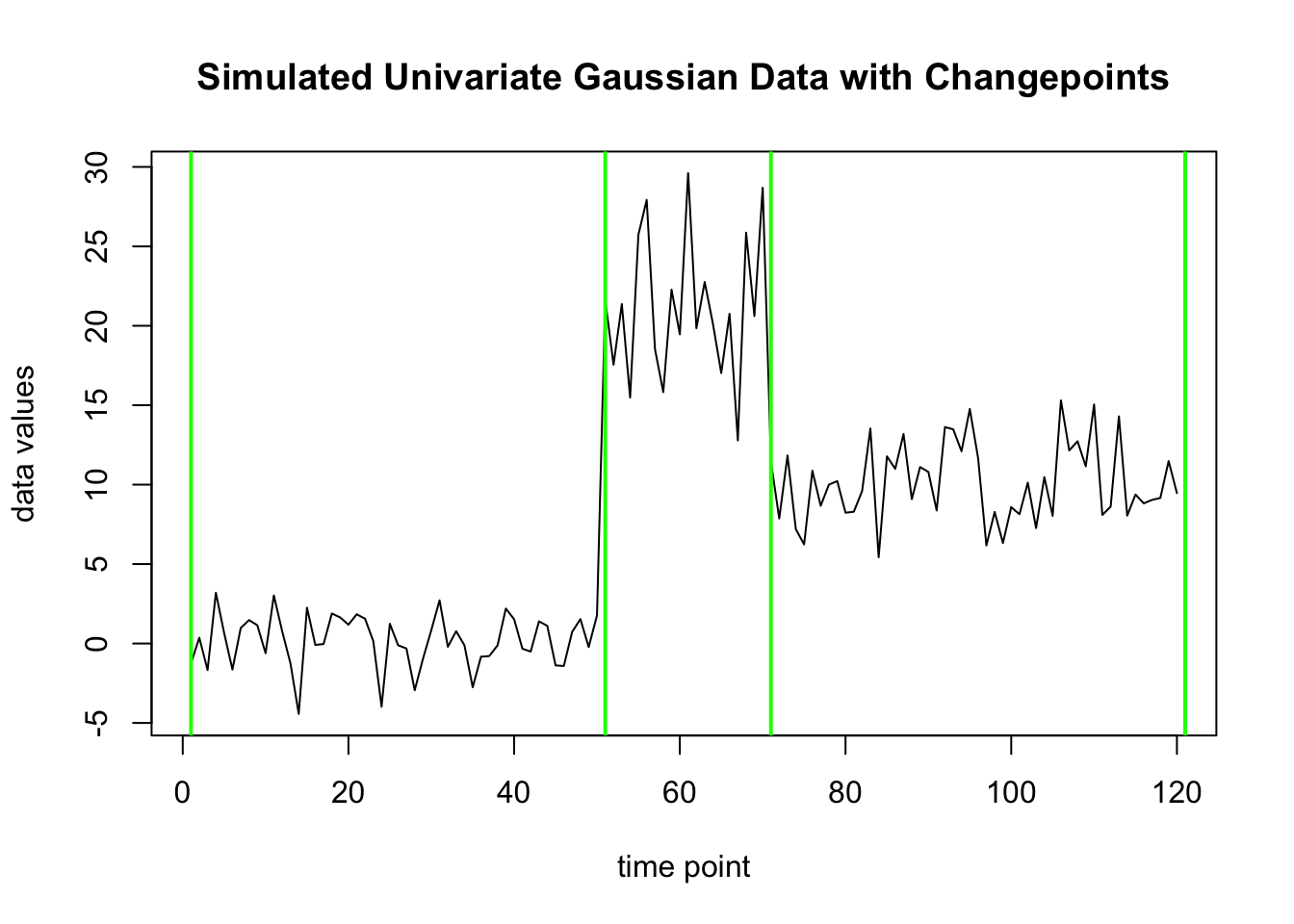This package “ocp” provides code implementing Bayesian online changepoint detection. This tutorial will show how to use the main functions in this package.

# 1 Running Online Bayesian Changepoint Detection

## 1.1 Generate Univariate Gaussian Data

The figure below shows the simulated univariate gaussian data used for this tutorial, and the locations of the true changepoints.

`````` # the true changepoint locations including the first and last point
truecps<- c(1, 51, 71, 121)
#simulate the data
set.seed(1)
uvg<- c(rnorm(n=diff(truecps), mean=0, sd=2),
rnorm(n=diff(truecps), mean=20, sd=4),
rnorm(n=diff(truecps), mean=10, sd=3))

# view the data
plot(uvg, main = "Simulated Univariate Gaussian Data with Changepoints",
ylab = "data values", xlab="time point", type= "l", col = "black", cex=0.5)
# show the changepoints on the graph
for(cp in truecps){
abline(v=cp, col = "green", lwd= 2)
}``````## 1.2 Run Basic Online Changepoint Detection

This section will run the basic onlineCPD function to output an ocpd object from the simulated data.

``````# running the basic function with all the default settings
ocpd1<- onlineCPD(uvg)

# view results
ocpd1
#>  "1 -variate data."
#>  "Attributes returned:"
#>   "R"                 "prevR"             "prevRprod"
#>   "prevRsum"          "prevDataPt"        "time"
#>   "ocpd_settings"     "threshcps"         "max"
#>  "update_paramsT"    "update_params0"    "init_params"
#>  "logprobmaxes"      "logprobcps"        "currmu"
#>  "changepoint_lists"
#>  "Changepoints:"
#> []
#>   1 51 71``````

Printing the results shows a list of the attributes returned, and the main finding which is the output list of changepoints.

Following is some explanation of each attribute returned.

### 1.2.1 Main Outputs

The main output from the OCPD objects are the changepoints list and the R matrix if the it is chosen to be returned.

• Changepoints List: list of changepoints computed from different approaches
• colmaxes: the list of changepoints taken from the maximum probability in each column of the R matrix
• threshchps: the list of changepoints that have run length probability above a threshold value, e.g. 0.5
• maxCPs: the list of changepoints with the highest overall probability

The list of possible changepoints can be accessed from the attribute “changepoint_lists”. The code and output from this example is shown below.

``````cpdf<- data.frame(method=names(ocpd1\$changepoint_lists))
cpdf\$changepoints<- unlist(ocpd1\$changepoint_lists, recursive = FALSE)
kable(cpdf)``````
method changepoints
colmaxes c(1, 51, 71)
threshcps c(1, 51, 71)
maxCPs c(1, 51, 71)

Additional outputs are also returned - these are needed for running the algorithm online since this algorithm can work being called point by point to build on a pre-existing ocpd object, but not needed for interpreting the final results. These outputs are:

• ocpd_settings: stores the settings used in running the ocpd function
• prevR: the column of the R matrix for the previous step
• prevRprod, prevRsum: versions of the R matrix containing cumulative product or sum along diagonals
• prevDataPt: the previous data point processed - needed for replacing missing data options
• time: the total number of points processed within this ocpd object
• update_paramsT: the current parameters used in the UPM update functions
• update_params0: the initial parameters used in the UPM update functions
• init_params: the params used to initialize the UMP update_params0
• logprobcps, logprobmaxes: the list of changepoints with the highest probability at each step, and the probability associated respectively
• currmu: the current mu compute after each timepoint

## 1.3 Online Changepoint Detection Function Options

This section will explain the various options possible to set when running the online changepoint detction function. The main choices to be made in running the function are:

• speed:
• Fastest method (\(O(n)\)): do not return any unnecessary information and truncate the R vector, set \(getR=FALSE\), \(optionalOutputs=FALSE\), \(truncRlim = 10^{(-4)}\)
• Running without truncation (\(O(n^2)\)): to prevent truncation, set \(truncRlim =0\)
• Returning the R matrix (\(O(n^3)\)): set \(getR=TRUE\) to end up storing the computed R matrix in the ocpd object
• truncation:
• truncation can be done by probability threshold (truncRlim)
• hard constraints on length can also be set: maxRlength (max length allowed before truncating), minRlength (min length required before starting to truncate)
• probability model:
• model choice specified by name or letter, e.g. \(probModel=list("g")\), or \(probModel=list("gaussian")\)
• the initialization settings for the probability model: \(init\_params=list(list(m=0, k=0.01, a=0.01, b=0.0001))\)
• mutlivariate: set \(multivariate=TRUE\) if the data is multivariate

The following sections show how to run the algorithm with various configurations.

### 1.3.1 Settings affecting speed: getR, truncRlim, optionalOutputs

The following code shows some examples how to run the function with different configurations.

``````# slowest mode: saving R matrix and optional outputs, no truncation
ocpd2<- onlineCPD(uvg, getR=TRUE, optionalOutputs = TRUE,
hazard_func=function(x, lambda){const_hazard(x, lambda=100)})

# plot the results, the R matrix will be visible
plot(ocpd2, cplistID = 3)``````